## Parabola Evolute

Given a Parabola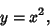(1)

the parametric equation and its derivatives are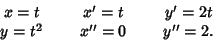(2)(3)

The Tangent Vector is(4)

so the parametric equations of the evolute are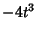(5)(6)

and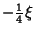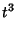(7)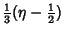(8)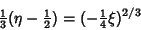(9)(10)

The Evolute is therefore(11)

This is known as Neile's Parabola and is a Semicubical Parabola. From a point above the evolute three normals can be drawn to the Parabola, while only one normal can be drawn to the Parabola from a point below the Evolute.